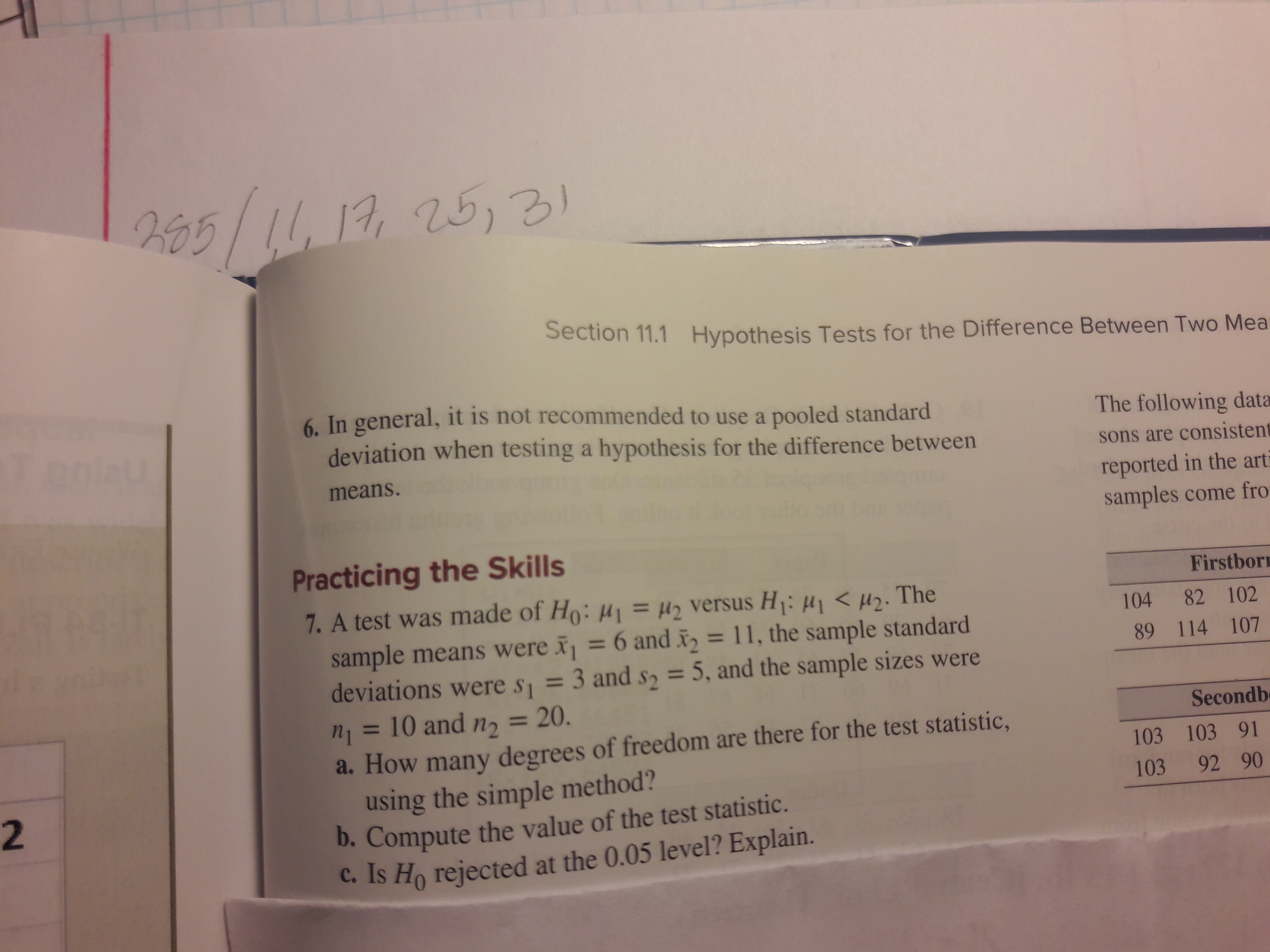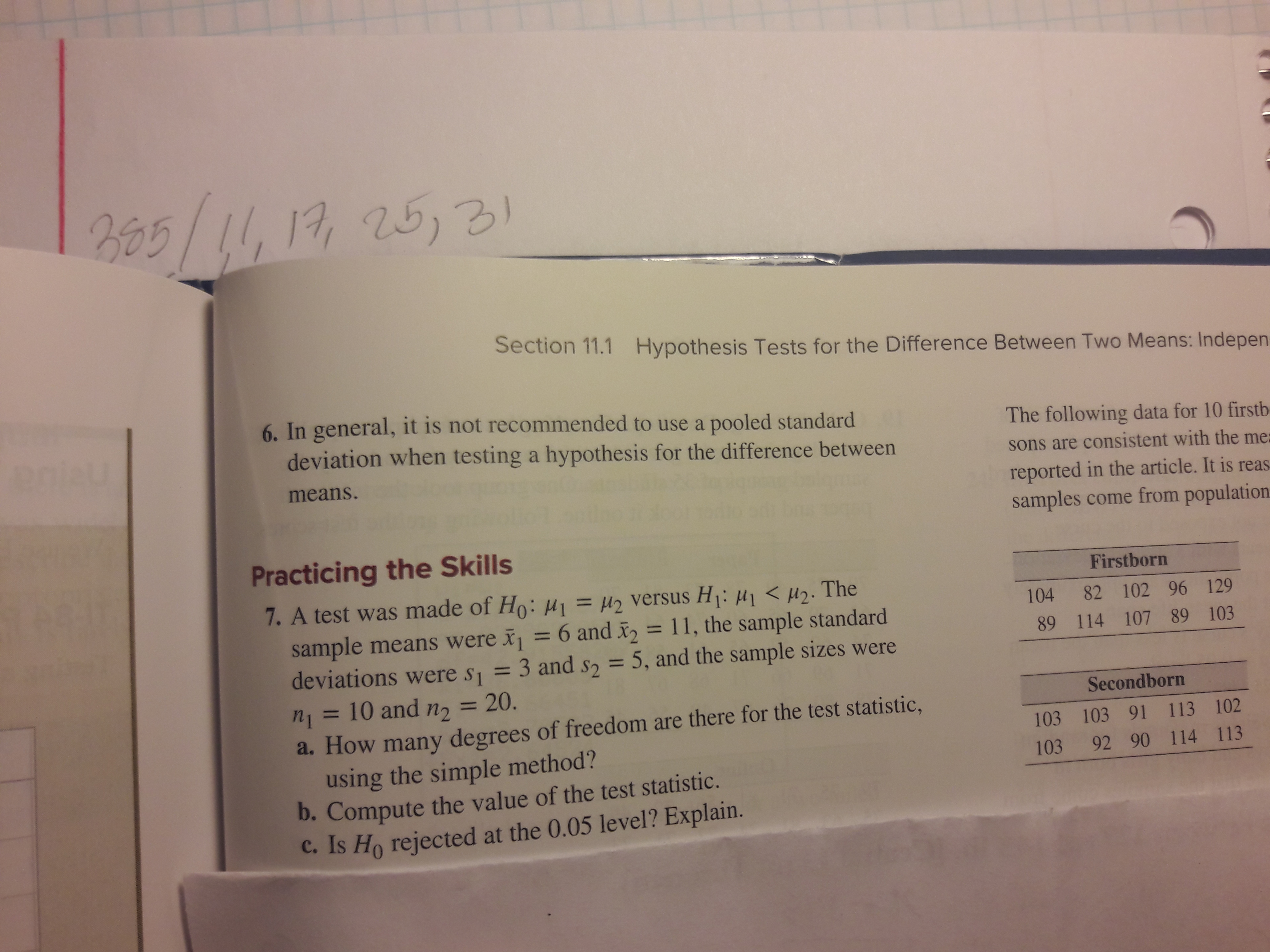# 1225,3Section 11.1 Hypothesis Tests for the Difference Between Two Mea-6 In general, it is not recommended to use a pooled standarddeviation when testing a hypothesis for the difference betweenThe following datasons are consistentmeans.reported in the artsamples come froPracticing the SkillsFirstbor7. A test was made of Ho: HI=42 versus H1: H1H2. The10482 102sample means were = 6 and i= 11, the sample standarddeviations were s = 3 ands = 5, and the sample sizes were10 and n 20.89 114 1071Secondba. How many degrees of freedom are there for the test statistic,using the simple method?D. Compute the value of the test statistic.C. Is Ho rejected at the 0.05 level? Explain.103 103 912103 92 90 Bo5/1,17 253Section 11.1Hypothesis Tests for the Difference Between Two Means: Indepenprieu6. In general, it is not recommended to use a pooled standarddeviation when testing a hypothesis for the difference betweenThe following data for 10 firstbsons are consistent with the memeans.reported in the article. It is reassamples come from populationPracticing the SkillsFirstborn7. A test was made of Ho: H2 versus Hi: 1H2.Thesample means were = 6 and 2= 11, the sample standarddeviations were s1 = 3 and s2 = 5, and the sample sizes were10482 102 96 12989 114 107 89 103n1= 10 and n = 20.Secondborna. How many degrees of freedom are there for the test statistic,using the simple method?b. Compute the value of the test statistic.C. Is Ho rejected at the 0.05 level? Explain.103 103 91 113 102103 92 90 114 113

Question
1 viewshelp_outlineImage Transcriptionclose12 25,3 Section 11.1 Hypothesis Tests for the Difference Between Two Mea- 6 In general, it is not recommended to use a pooled standard deviation when testing a hypothesis for the difference between The following data sons are consistent means. reported in the art samples come fro Practicing the Skills Firstbor 7. A test was made of Ho: HI=42 versus H1: H1H2. The 104 82 102 sample means were = 6 and i= 11, the sample standard deviations were s = 3 ands = 5, and the sample sizes were 10 and n 20. 89 114 107 1 Secondb a. How many degrees of freedom are there for the test statistic, using the simple method? D. Compute the value of the test statistic. C. Is Ho rejected at the 0.05 level? Explain. 103 103 91 2 103 92 90 fullscreenhelp_outlineImage TranscriptioncloseBo5/1,17 253 Section 11.1Hypothesis Tests for the Difference Between Two Means: Indepen prieu 6. In general, it is not recommended to use a pooled standard deviation when testing a hypothesis for the difference between The following data for 10 firstb sons are consistent with the me means. reported in the article. It is reas samples come from population Practicing the Skills Firstborn 7. A test was made of Ho: H2 versus Hi: 1H2.The sample means were = 6 and 2= 11, the sample standard deviations were s1 = 3 and s2 = 5, and the sample sizes were 104 82 102 96 129 89 114 107 89 103 n1= 10 and n = 20. Secondborn a. How many degrees of freedom are there for the test statistic, using the simple method? b. Compute the value of the test statistic. C. Is Ho rejected at the 0.05 level? Explain. 103 103 91 113 102 103 92 90 114 113 fullscreen
check_circle

Step 1

(a)

The degrees of freedom is 28, which is obtained as follows:

Step 2

(b)

Pooled estimate:

The pooled estimate of the population variance is,

Step 3

The test statistic is –2.90, which i...

### Want to see the full answer?

See Solution

#### Want to see this answer and more?

Solutions are written by subject experts who are available 24/7. Questions are typically answered within 1 hour.*

See Solution
*Response times may vary by subject and question.
Tagged in

### Statistics